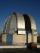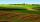# Area of the cone

Calculate the surface area of the cone, you know the base diameter 25 cm and a height 40 cm.

Result

S =  2136.583 cm2

#### Solution:Leave us a comment of example and its solution (i.e. if it is still somewhat unclear...):Be the first to comment!#### To solve this example are needed these knowledge from mathematics:

Pythagorean theorem is the base for the right triangle calculator.

## Next similar examples:

1. Rotating cone IICalculate area of surface of rotating cone with base radius r=19 cm and height h=9 cm.
2. CylinderThe cylinder surface is 859 dm2, its height is equal to the radius of the base. Calculate height of this cylinder.
3. ObservatoryObservatory dome has the shape of a hemisphere with a diameter d = 10 m. Calculate the surface.
4. GreenhouseGarden plastic greenhouse is shaped half cylinder with a diameter of 6 m and base length 20 m. At least how many m2 of plastic is need to its cover?
5. PipeHow long is the pipe with an outside diameter of 1.44 m if his coloring consumed 44 kg of color. 1 kg of color coverage is 9 m2.Alaska is the largest state in the United States and has a surface area of approximately 588,000 square miles. Montana has a surface area that is approximately 25% of the surface area of Alaska. What is the approximate surface area of Montana?
7. GardenThe garden around the new majer is divided as follows: 35% vegetable, 30% fruit orchards, 10% flowers and the remaining 120 m2 are lawns. What is the total area of the garden?
8. Land areasTwo land areas is 244 m2. The first parcel is 40 m2 less than twice of the second one. What have acreage of each parcel?
9. Cone 15The radius of the base of a right circular cone is 14 inches and it's height 18 inches. What is the slant height?
10. Four ropesTV transmitter is anchored at a height of 44 meters by four ropes. Each rope is attached at a distance of 55 meters from the heel of the TV transmitter. Calculate how many meters of rope were used in the construction of the transmitter. At each attachment.
11. Chord circleThe circle to the (S, r = 8 cm) are different points A, B connected segment /AB/ = 12 cm. AB mark the middle of S'. Calculate |SS'|. Make the sketch.
12. Broken treeThe tree was 35 meters high. The tree broke at a height of 10 m above the ground. Top but does not fall off it refuted on the ground. How far from the base of the tree lay its peak?
13. WindbreakA tree at a height of 3 meters broke in the windbreak. Its peak fell 4.5 m from the tree. How tall was the tree?
14. CandyPeter had a sachet of candy. He wanted to share with his friends. If he gave them 30 candies, he would have 62 candies. If he gave them 40 candies, he would miss 8 candies. How many friends did Peter have?
15. EnrollmentThe enrollment at a local college increased 4% over last year's enrollment of 8548. Find the increase in enrollment (x1) and the current enrollment (x2).
16. ShotsDetermine the percentage rate of keeper interventions if from 32 shots doesn't caught four shots.
17. Divisible by 5How many three-digit odd numbers divisible by 5, which are in place ten's number 3?